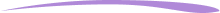# What is a Product in Math?

A product in math is the result we obtain when we multiply two or more numbers together.Author
Michelle GriczikaPublished
September 13, 2023# What is a product in math?

A product in math is the result we obtain when we multiply two or more numbers together.Author
Michelle GriczikaPublished
September 13, 2023# What is a Product in Math?

A product in math is the result we obtain when we multiply two or more numbers together.Author
Michelle GriczikaPublished
September 13, 2023Key takeaways

• The product meaning in math refers to the result achieved when two or more numbers, known as factors, are multiplied together. It is a fundamental concept in many areas of mathematics.
• Calculating a product is straightforward, involving multiplying the numbers together, whether they are whole numbers, fractions, decimals, or even include variables.
• The understanding of the concept of the product extends beyond math assignments. It is practical in daily life, from calculating the prices of multiple items to figuring out the area of a room.

Understanding the language of mathematics can sometimes feel like learning a new language altogether for both students and parents alike. The term product often comes up in math lessons and homework. But what is a product in math? We’ll explain what a product is, show how to find it, and provide practice problems to help reinforce the concept.

## Definition of product in mathSo, what is “product” in math? In mathematics, product means the result you get after multiplying numbers together. The numbers that are being multiplied are known as factors. So, the final result is called the product when we multiply factors, whether they’re whole numbers, fractions, or even decimals. This concept is at the heart of many areas in mathematics, which is why it’s so important to understand the products definition.

## How to find the product in mathFiguring out the product in math is simple: you multiply the numbers. The process can vary from elementary multiplication problems, such as 2 x 3, to more complex ones involving bigger numbers or decimals. Regardless of the complexity, the answer derived from the multiplication operation is called a product – and yes, this holds even when variables are in the equation.

But what happens when there are more than two numbers or factors involved? Do not fret! The method remains the same when wondering, “what’s product in math?”.

Consider a simple example: To find the product of 2, 3, and 4, multiply them in any order. You can multiply 2 and 3 first to get 6, then multiply 6 by 4 to get 24. Alternatively, you can multiply 4 and 2 first to get 8, then multiply 8 by 3 to get 24. This flexibility is due to the commutative property of multiplication, which states that the order of numbers does not change the product.

It’s also important to remember that the math product of any number and zero is always zero. This is known as the zero property of multiplication.

When dealing with fractions or decimals, the process remains essentially the same. You multiply the fractions or the decimals directly. However, calculating these might require additional steps or a good grasp of fraction and decimal operations.

Lastly, multiplication and its product have a wide range of applications in real-world scenarios, from calculating the prices of multiple items to figuring out the area of a room. Therefore, understanding the concept of a product is not just crucial for math assignments but also practical in daily life.

Why is understanding the concept of 'product' important?

Understanding the concept of ‘product’ is a foundational skill in mathematics. Being familiar with it can make more advanced math topics easier to understand.## Practice problems

If your child is having difficulty, using our math practice app to help reinforce these concepts might be beneficial. Consistent practice makes perfect!

## FAQs about products in mathA little blurb of text about why we crafted these really helpful FAQs for you!

In mathematics, ‘product’ refers to the result of multiplying numbers together. For example, if you multiply 3 and 4, the product is 12.

So, what is the product in math?  To find the product in math, you perform multiplication. This could involve two or more numbers. For example, the product of 5 and 6 is 30 because 5 multiplied by 6 equals 30.

Yes, the term ‘product’ is specifically used to denote the result of a multiplication operation. It is not used in addition, subtraction, or division.

In a multiplication operation, the numbers being multiplied are called ‘factors,’ while the result of the multiplication is called the ‘product.’ For example, in 6 * 4 = 24, 6 and 4 are factors, and 24 is the product.Lesson creditsMichelle Griczika

Michelle Griczika is a seasoned educator and experienced freelance writer. Her years teaching first and fifth grades coupled with her double certification in elementary and early childhood education lend depth to her understanding of diverse learning stages. Michelle enjoys running in her free time and undertaking home projects.

Michelle Griczika

Michelle Griczika is a seasoned educator and experienced freelance writer. Her years teaching first and fifth grades coupled with her double certification in elementary and early childhood education lend depth to her understanding of diverse learning stages. Michelle enjoys running in her free time and undertaking home projects.# Are you a parent, teacher or student?

Are you a parent or teacher?

## Hi there!

Book a chat with our team